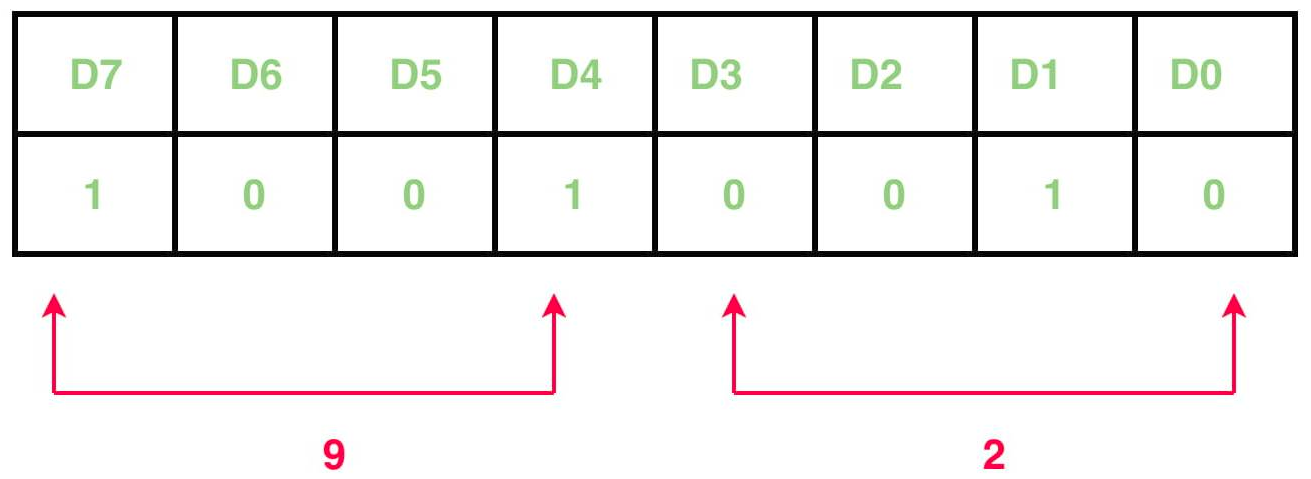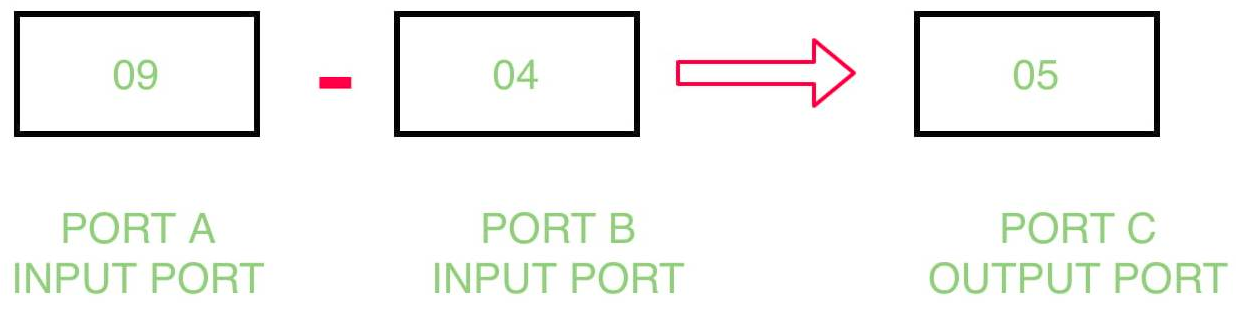# Subtract content of two ports by interfacing 8255 with 8085 microprocessor

Problem – Write an assembly program which determine the subtraction of contents of port B from port A and store the result in port C by interfacing 8255 with 8085 microprocessor.

Example –Algorithm –

1. Construct the control word register
2. Input the data from port A and port B
3. Subtract the contents of port A and port B
4. Display the result in port C
5. Halt the program

Program –

MVI A, 92 A <- 92
OUT 83 Control Register <- A
IN 81 A <- Port B
MOV B, A B <- A
IN 80 A <- Port A
SUB B A <- A – B
OUT 82 Port C <- A
RET Return

Explanation –

1. MVI A, 92: means that the value of control register is 92.
```D7=1         I/O mode
D6=0 & D5=0  Port A is in mode 0
D4=1         Port A is taking input
D3=0 & D0=0  Port C is not taking part
D2=0         Port B is in mode 0
D1=1         Port B is taking input
```
2. OUT 83: putting the value of A in 83H which is the port number of port control register.
3. IN 81: take input from 81H which is the port number of port B.
4. MOV B, A: copies the content of A register to B register.
5. IN 80: taking input from 80H which is the port number of port A.
6. SUB B: subtract the contents of A register and B register.
7. OUT 82: display the result in 81H which is the port number of port C.
8. RET: return

Attention reader! Don’t stop learning now. Get hold of all the important CS Theory concepts for SDE interviews with the CS Theory Course at a student-friendly price and become industry ready.

My Personal Notes arrow_drop_upCheck out this Author's contributed articles.

If you like GeeksforGeeks and would like to contribute, you can also write an article using contribute.geeksforgeeks.org or mail your article to contribute@geeksforgeeks.org. See your article appearing on the GeeksforGeeks main page and help other Geeks.

Please Improve this article if you find anything incorrect by clicking on the "Improve Article" button below.